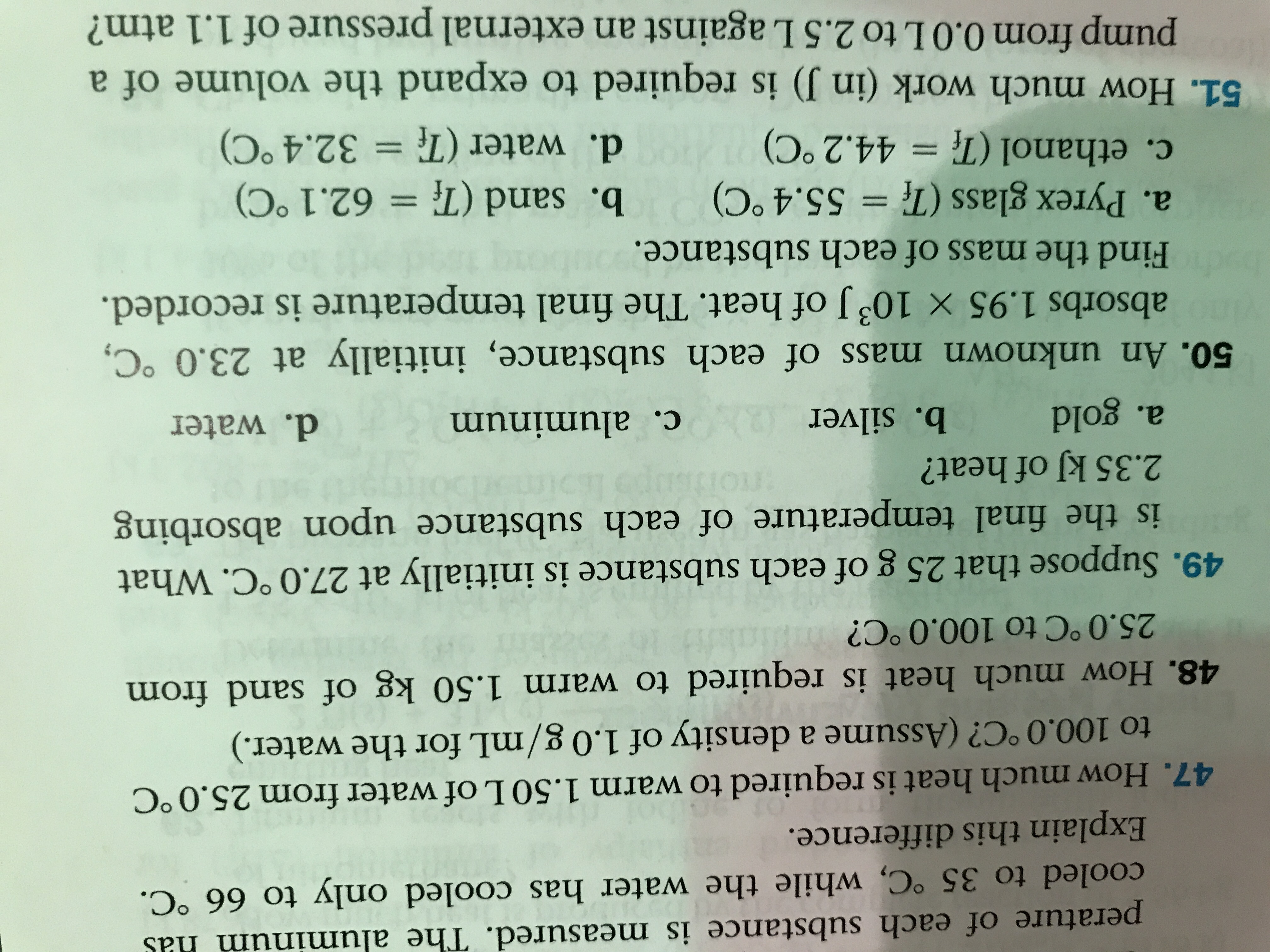# m hasperature of each substance is measured. The aluminurcooled to 35 °C, while the water has cooled only to 66 °C.Explain this difference.47. How much heat is required to warm 1.50 L of water from 25.0 °Cto 100.0 °C? (Assume a density of 1.0 g/mL for the water.)48. How much heat is required to warm 1.50 kg of sand from25.0 °C to 100.0 °C?49. Suppose that 25 g of each substance is initially at 27.0 °C. Whatis the final temperature of each substance upon absorbing2.35 kJ of heat?a. goldb. silverc. aluminumd. water50. An unknown mass of each substance, initially at 23.0 °C,absorbs 1.95 × 103 J of heat. The final temperature is recorded.Find the mass of each substance.a. Pyrex glass (T = 55.4°C) b. sand (T = 62.1°C)c. ethanol (T= 44.2°C)d. water (T = 32.4°C)51. How much work (in J) is required to expand the volume of apump from 0.0L to 2.5 Lagainst an external pressure of 1.1 atm?

Question
58 views

Question 49a, b, c, dhelp_outlineImage Transcriptionclosem has perature of each substance is measured. The aluminur cooled to 35 °C, while the water has cooled only to 66 °C. Explain this difference. 47. How much heat is required to warm 1.50 L of water from 25.0 °C to 100.0 °C? (Assume a density of 1.0 g/mL for the water.) 48. How much heat is required to warm 1.50 kg of sand from 25.0 °C to 100.0 °C? 49. Suppose that 25 g of each substance is initially at 27.0 °C. What is the final temperature of each substance upon absorbing 2.35 kJ of heat? a. gold b. silver c. aluminum d. water 50. An unknown mass of each substance, initially at 23.0 °C, absorbs 1.95 × 103 J of heat. The final temperature is recorded. Find the mass of each substance. a. Pyrex glass (T = 55.4°C) b. sand (T = 62.1°C) c. ethanol (T= 44.2°C) d. water (T = 32.4°C) 51. How much work (in J) is required to expand the volume of a pump from 0.0L to 2.5 Lagainst an external pressure of 1.1 atm? fullscreen
check_circle

Step 1

The relationship between heat, mass, specific heat and change in temperature is given by,

Step 2

Rearrange the above equation.

Step 3

a. Gold:

The specific heat capacity of gold is 0.129 J/g˚C

Calculate ...

### Want to see the full answer?

See Solution

#### Want to see this answer and more?

Solutions are written by subject experts who are available 24/7. Questions are typically answered within 1 hour.*

See Solution
*Response times may vary by subject and question.
Tagged in

### Physical Chemistry